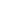Weekly Boost

Week 14Slope and Spelling

Learners will practice finding slope from the (x, y) coordinates of two points using the slope formula. Then, given a variety of nature-themed sentences, they'll pay close attention to the context of each sentence to identify and correct the spelling errors.
Students will practice finding slope from the (x, y) coordinates of two points using the slope formula. Then, given a variety of nature-themed sentences, they'll pay close attention to the context of each sentence to identify and correct the spelling errors.
Finding Slope From Two Points
Worksheet
Finding Slope From Two Points
This eighth-grade algebra worksheet gives students a chance to practice finding the slope from two points using the slope formula.
Math
Worksheet
Spelling With Plurals and Possessives
Worksheet
Spelling With Plurals and Possessives
Practice spelling with plurals and possessives in this grammar worksheet for middle grades!
WorksheetSend home these fun free resources to help reinforce the skills you're teaching in the classroom.